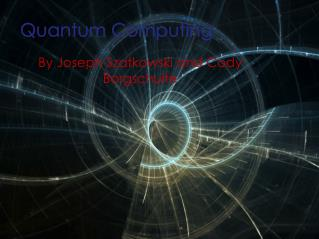# Quantum Computing - PowerPoint PPT PresentationDownload PresentationQuantum Computing

Quantum Computing
Download Presentation## Quantum Computing

- - - - - - - - - - - - - - - - - - - - - - - - - - - E N D - - - - - - - - - - - - - - - - - - - - - - - - - - -
##### Presentation Transcript

1. Quantum Computing By Joseph Szatkowski and Cody Borgschulte

2. What is a Quantum Computer? Uses phenomenon associated with quantum mechanics instead of electrical circuitry Quantum mechanics explains how particles interact on an individual level. Superposition Entanglement

3. Qubits Uses qubits instead of bits Unlike bits, qubits can be on, off, or a superposition of both. 2 qubits can hold 00, 01, 10, 11, or any superposition of these values. This allows a quantum computer to perform multiple calculations simultaneously.

4. Physical representation A qubit can be represented by a single electron. Electrons have a property called spin, which determines how they act in a magnetic field. Up spin and down spin representing 1 and 0

5. Superposition Quantum particles have the ability to exist partially in different states. When measured the superposition collapses into a single state. A superposition can be represented by a complex number, with coefficients representing how much of each state there is.

6. Entanglement Entanglement allows two particle to interact directly with each other, allowing operations to be performed. Necessary because particles cannot be observed during calculations as this would collapse the superposition.

7. History First theorized by Paul Benioff in 1981 In 1998 the scientists at Los Alamos created an extremely simple prototype using 1 qubit. In 2000 a 7 qubit computer was created. This computer was programmed using radio frequency pulses. In 2001 Shor's algorithm was successfully demonstrated. In 2007 D-Wave used a 16 qubit computer to solve a Sudoku puzzle.

8. Limitations To create a quantum computer you must be able to control and measure particles. Lasers, superconductors, etc. Super expensive It is unlikely quantum computers will be publicly available any time soon. Cannot measure while calculating. Individual operations are slower.

9. Applications Quantum computer can perform algorithms which transistor computers can't. Shor's algorithm can be used to factor large numbers in polynomial time (O((log N)^3)). Can be used to break RSA codes Can simulate quantum mechanics. Study cures, analyze large networks, solve other “unsolvable” problems.

10. More Applications! • NAS Ames Research Center • Exascale Computing (10^18 floating point operations per second) • Grover’s algorithm (N^(1/2))

11. Questions?